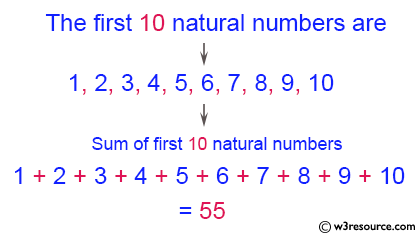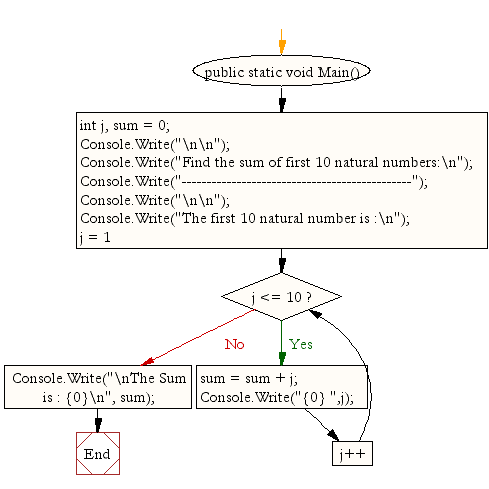﻿ C# - Display the sum of first 10 natural numbers# C# Sharp Exercises: Display the sum of first 10 natural numbers

## C# Sharp For Loop: Exercise-2 with Solution

Write a C# Sharp program to find the sum of first 10 natural numbers.

Pictorial Presentation:Sample Solution:

C# Sharp Code:

``````using System;
public class Exercise2
{
public static void Main()
{
int  j, sum = 0;

Console.Write("\n\n");
Console.Write("Find the sum of first 10 natural numbers:\n");
Console.Write("----------------------------------------------");
Console.Write("\n\n");

Console.Write("The first 10 natural number are :\n");
for (j = 1; j <= 10; j++)
{
sum = sum + j;
Console.Write("{0} ",j);
}
Console.Write("\nThe Sum is : {0}\n", sum);
}
}
```
```

Sample Output:

```Find the sum of first 10 natural numbers:
----------------------------------------------

The first 10 natural number are :
1 2 3 4 5 6 7 8 9 10
The Sum is : 55
```

Flowchart:C# Sharp Code Editor:

Contribute your code and comments through Disqus.

What is the difficulty level of this exercise?

Test your Programming skills with w3resource's quiz.

﻿